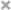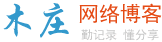# javascript 没有类吗JavaScript 是基于对象，但不完全面向对象的编程语言。在 JS 面向对象的编程模式中，有两个核心概念： 对象和类。在 ECMAScript6 规范之前，JavaScript 没有类的概念，仅允许通过构造函数来模拟类，通过原型实现继承。

## 1 类声明

 1234 `// 类声明``class Student {}``// 类表达式``const Student = class {}`

## 2 为什么说它是语法糖

 12345678910111213 `class Student {``    ``take() {}``}``const a = ``new` `Student()``console.log(``typeof` `Student) ``// function``console.log(a.take === Student.prototype.take) ``// true` `// 同等于``function` `Student() {}``Student.prototype.take = ``function``() {}``const a = ``new` `Student()``console.log(``typeof` `Student) ``// function``console.log(a.take === Student.prototype.take) ``// true`

## 3 类包含的属性和方法

 123456789101112131415161718192021 `class Student {``    ``// 实例属性 也可以放在这``    ``// b = 1``    ``// 静态属性``    ``static a = 1``    ``// 构造函数``    ``constructor() {``        ``// 实例属性 - 也可以放在类的最顶层``        ``this``.b = 1``    ``}``    ``// 获取函数``    ``get myName() {}``    ``// 设置函数``    ``set myName() {}``    ``// 静态方法 不会被实例继承``    ``static show() {}``    ``// 方法``    ``take() {}``    ``// 私有方法``    ``_take() {}``}`

3.1 类的构造函数

 1234567 `class A {``    ``constructor() {``        ``this``.name = ``'小明'``    ``}``}``const b = ``new` `A()``b.constructor === A.prototype.constructor ``// true`

3.2 类的方法

 1234 `class Student {``    ``// 方法``    ``take() {}``}`

3.3 类的静态方法

 12345678910 `class A {``    ``// 静态方法``    ``static show() {``        ``console.log(``'hi'``)``    ``}``}``class B extends A {}``const c = ``new` `A()``c.show() ``// c.show is not a function``B.show() ``// hi`

3.4 类的私有方法

es6中没有提供这个方法，但是通常都是在方法前面加上下划线来表示。

 123456 `class A {``    ``// 私有方法``    ``_show() {``        ``console.log(``'hi'``)``    ``}``}`

3.5 取值函数（getter）和存值函数（setter）

 1234567891011121314 `class A {``  ``constructor () {``    ``this``.name = ``'小米'``  ``}``  ``get name () {``    ``return` `'get'``  ``}``  ``set name (val) {``    ``console.log(``'set'` `+ val)``  ``}``}``const b = ``new` `A()``b.name ``// get``b.name = 123 ``// set123`

## 4 类的继承

4.1 通过extends实现继承

 1234567 `class A {``    ``// 静态方法``    ``static show() {``    ``console.log(``'hi'``)``  ``}``}``class B extends A {}`

4.2 super方法

 1234567891011121314 `class A {``  ``constructor () {``    ``this``.name = ``'小米'``  ``}``    ``show() {``    ``console.log(``'hi'``)``  ``}``}``class B extends A {``  ``constructor () {``    ``super``() ``// 如果不写super，则会报ReferenceError错误``  ``}``}``const c = ``new` `B()`

super方法中也可以传参

 123456789101112131415 `class A {``  ``constructor (name) {``    ``this``.name = name``  ``}``    ``show() {``    ``console.log(``'hi'``)``  ``}``}``class B extends A {``  ``constructor () {``    ``super``(``'小红'``)``  ``}``}``const c = ``new` `B()``c.name ``// 小红`

## 5 其他

5.1 方法中的this指向

 1234567891011 `class A {``  ``constructor () {``    ``this``.name = ``'小米'``  ``}``  ``show () {``    ``console.log(``this``.name)``  ``}``}``const b = ``new` `A()``b.show() ``// 小米``const { show } = b ``// Cannot read property 'name' of undefined`

1、在构造函数中绑定this

 123456789 `class A {``  ``constructor () {``    ``this``.name = ``'小米'``    ``this``.show = ``this``.show.bind(``this``)``  ``}``  ``show () {``    ``console.log(``this``.name)``  ``}``}`

2、使用箭头函数

 123456789 `class A {``  ``constructor () {``    ``this``.name = ``'小米'``    ``this``.show = () => ``this``  ``}``  ``show () {``    ``console.log(``this``.name)``  ``}``}`

5.2 区分是否继承了这个类

 12345678910111213141516 `class A {``  ``constructor () {``    ``this``.name = ``'小米'``  ``}``    ``show() {``    ``console.log(``'hi'``)``  ``}``}``class B extends A {``  ``constructor () {``    ``super``()``  ``}``}``class C {}``Object.getPrototypeOf(B) === A ``// true 是继承的A类``Object.getPrototypeOf(B) === C ``// false 没有继承C类`

【推荐学习：javascript高级教程

javascript怎么删除dom

es6中新的变量声明方式介绍（附代码）

javascript是h5吗

javascript如何设置焦点移除

javascript创建元素的方法（代码）

js怎么格式化日期

javascript如何定义变量

h5的视频播放库video.js详解

javascript中substr和substring的区别是什么

javascript和css的区别是什么### 评论

• 欢迎访问木庄网络博客
• 可复制：代码框内的文字。
• 方法：Ctrl+C。